Students can Download Maths Chapter 2 Percentage and Simple Interest Ex 2.1 Questions and Answers, Notes Pdf, Samacheer Kalvi 7th Maths Book Solutions Guide Pdf helps you to revise the complete Tamilnadu State Board New Syllabus and score more marks in your examinations.

Tamilnadu Samacheer Kalvi 7th Maths Solutions Term 3 Chapter 2 Percentage and Simple Interest Ex 2.1

Question 1.
In each of the following grid, find the numbers of coloured squares and express it as a fraction, decimal and percentage.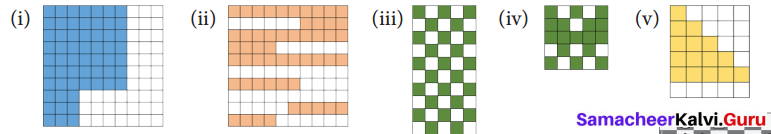Solution:
Number of coloured square = 58
Total number of squares = 100
∴ Fraction : $$\frac { 58 }{ 100 }$$
Decimal : 0.58
Percentage : 58%

(ii) Number of coloured square = 53
Total number of squares = 100
∴ Fraction : $$\frac { 53 }{ 100 }$$
Decimal : 0.53
Percentage : 53%

(iii) Number of coloured square = 25
Total number of squares = 50
∴ Fraction : $$\frac { 25 }{ 50 }$$

Decimal : $$\frac { 25 }{ 50 }$$ × $$\frac { 2 }{ 2 }$$
= $$\frac { 50 }{ 100 }$$
= 0.50

Percentage : $$\frac { 25 }{ 50 }$$ × $$\frac { 100 }{ 100 }$$
= $$\frac { 25 }{ 50 }$$ × 100% = 50%(iv) Number of coloured square = 17
Total number of squares = 25
∴ Fraction : $$\frac { 17 }{ 25 }$$
Decimal : $$\frac { 17 }{ 25 }$$ × $$\frac { 4 }{ 4 }$$
= $$\frac { 68 }{ 100 }$$ = 0.68
Percentage : $$\frac { 17 }{ 25 }$$ × $$\frac { 100 }{ 100 }$$
= $$\frac { 17 }{ 25 }$$ × 100%
= 68%

(v) Number of coloured square = 15
Total number of squares = 30
∴ Fraction : $$\frac { 15 }{ 30 }$$
Decimal : $$\frac { 15 }{ 30 }$$
= $$\frac { 1 }{ 2 }$$ × $$\frac { 50 }{ 50 }$$
= $$\frac { 50 }{ 100 }$$ = 0.50
Percentage : $$\frac { 15 }{ 30 }$$
= $$\frac { 15 }{ 30 }$$ × $$\frac { 100 }{ 100 }$$
= $$\frac { 15 }{ 30 }$$ × 100%
= 50 %Question 2.
A picture of chess board is given.
(i) Find the percentage of the white coloured squares
(ii) Find the percentage of gray coloured squares
(iii) Find the percentage of the squares that have the pieces and
(iv) The squares that do not have the pieces.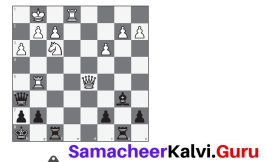Solution:
(i) Total number of squares in the chess board = 64
Number of white coloured squares = 32
Percentage = $$\frac { 32 }{ 64 }$$ × $$\frac { 100 }{ 100 }$$
= $$\frac { 32 }{ 64 }$$ × 100 %
= 50%

(ii) Grey coloured squares = 64
Percentage = $$\frac { 32 }{ 64 }$$ × $$\frac { 100 }{ 100 }$$
= $$\frac { 32 }{ 64 }$$ } [/latex] × 100 %
= 50 %

(iii) Number of squares having pieces = 20
Total number of squares = 64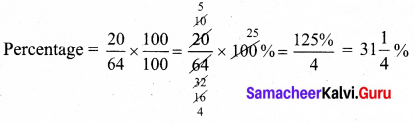(iv) Number of squares do not have pieces = 44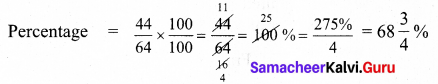Question 3.
A picture of dart board is given. Find the percentage of white coloured portion and black coloured portion.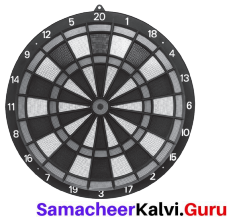Solution:
Total sector = 20
White coloured sector = 10
Black coloured sector = 10
Percentage of white : $$\frac { 10 }{ 20 }$$ × $$\frac { 100 }{ 100 }$$
Decimal : $$\frac { 10 }{ 20 }$$ × 100 %
= 50 %
Percentage of black colour : $$\frac { 10 }{ 20 }$$ × $$\frac { 100 }{ 100 }$$
Decimal : $$\frac { 10 }{ 20 }$$ × 100 %
= 50 %Question 4.
Write each of the following fraction as percentage.
(i) $$\frac { 36 }{ 50 }$$
(ii) $$\frac { 81 }{ 30 }$$
(iii) $$\frac { 42 }{ 56 }$$
(iv) 2 $$\frac { 1 }{ 4 }$$
(v) 1 $$\frac { 3 }{ 5 }$$
Solution:
(i) $$\frac { 36 }{ 50 }$$
= $$\frac { 36 }{ 50 }$$ × $$\frac { 100 }{ 100 }$$
= $$\frac { 36 }{ 50 }$$ × 100 %
= 72 %

(ii) $$\frac { 81 }{ 30 }$$
= $$\frac { 81 }{ 30 }$$ × $$\frac { 100 }{ 100 }$$
= $$\frac { 81 }{ 30 }$$ × 100 %
= 270 %

(iii) $$\frac { 42 }{ 56 }$$
= $$\frac { 42 }{ 56 }$$ × $$\frac { 100 }{ 100 }$$
= $$\frac { 42 }{ 56 }$$ × 100 %
= $$\frac { 21 }{ 28 }$$ × 100 %
= 75 %

(iv) 2 $$\frac { 1 }{ 4 }$$
= $$\frac { 9 }{ 4 }$$
= $$\frac { 9 }{ 4 }$$ × $$\frac { 100 }{ 100 }$$
= $$\frac { 9 }{ 4 }$$ × 100 %
= 225 %

(v) 1 $$\frac { 3 }{ 5 }$$
= $$\frac { 8 }{ 5 }$$
= $$\frac { 8 }{ 5 }$$ × $$\frac { 100 }{ 100 }$$
= $$\frac { 8 }{ 5 }$$ × 100 %
= 160 %Question 5.
Anbu scored 436 marks out of 500 in his exams. What was the percentage he scored?
Total marks = 500
Anbu’s Score = 436
Percentage = $$\frac { 436 }{ 500 }$$ × $$\frac { 100 }{ 100 }$$
= $$\frac { 436 }{ 500 }$$ × 100 %
= 87.2 %
Anbu’s Score = 87.2 %Question 6.
Write each of the following percentage as fraction,
(i) 21%
(ii) 93.1 %
(iii) 151 %
(iv) 65 %
(v) 0.64 %
Solution:
(i) 21%
= $$\frac { 21 }{ 100 }$$

(ii) 93.1 %
= $$\frac { 93.1 }{ 100 }$$
= $$\frac{93.1 \times 10}{100 \times 10}$$
= $$\frac { 931 }{ 1000 }$$

(iii) 151 %
= $$\frac { 151 }{ 100 }$$

(iv) 65 %
= $$\frac { 65 }{ 100 }$$
= $$\frac { 13 }{ 20 }$$

(v) 0.64 %
= $$\frac { 0.64 }{ 100 }$$
= $$\frac{0.64 \times 100}{100 \times 100}$$
= $$\frac { 64 }{ 10000 }$$
= $$\frac { 4 }{ 625 }$$

Question 7.
Iniyan bought 5 dozen eggs. Out of that 5 dozen eggs, 10 eggs are rotten. Express the number of good eggs as percentage.
Solution:
1 dozen eggs = 12
5 dozen = 5 × 12
Total eggs = 60 eggs
Rotten eggs = 10 Good
eggs = 60 – 10 = 50
Fraction of good eggs = $$\frac { 50 }{ 60 }$$
Percentage of good eggs = $$\frac { 50 }{ 60 }$$ × $$\frac { 100 }{ 100 }$$
= $$\frac { 50 }{ 60 }$$ × 100 %
= $$\frac { 5 }{ 6 }$$ × 100 %
= 83.33 %
Percentage of good eggs = 83.33 %Question 8.
In an election, Candidate X secured 48% of votes. What fraction will represent his votes?
Solution:
Percentage of votes x secured = 48% = $$\frac { 48 }{ 100 }$$
Fraction of votes x secured = $$\frac { 12 }{ 25 }$$

Question 9.
Ranjith total income was ₹ 7,500. He saved 25% of his total income. Find the amount saved by him.
Solution:
Total income of Ranjith = ₹ 7500
His savings = 25 % of 7500
= $$\frac { 25 }{ 100 }$$ of 7500
= $$\frac { 25 }{ 100 }$$ × 7500
= ₹ 1,875
∴ Amount saved by Ranjith = ₹ 1,875

Objective Type Questions

Question 1.
Thendral saved one fourth of her salary. Her savings percentage is
(i) $$\frac { 3 }{ 4 }$$
(ii) $$\frac { 1 }{ 4 }$$ %
(iii) 25 %
(iv) 1 %
Hint: $$\frac { 1 }{ 4 }$$ × $$\frac { 100 }{ 100 }$$
= $$\frac { 1 }{ 4 }$$ × 100 %
= 25 %
(iii) 25 %

Question 2.
Kavin scored 15 out of 25 in a test. The percentage of his marks is
(i) 60%
(ii) 15%
(iii) 25%
(iv) 15/25
Hint: $$\frac { 15 }{ 25 }$$ × $$\frac { 100 }{ 100 }$$
= $$\frac { 15 }{ 25 }$$ × 100 %
= 60 %
(i) 60%Question 3.
0.07% is
(i) $$\frac { 7 }{ 10 }$$
(ii) $$\frac { 7 }{ 100 }$$
(iii) $$\frac { 7 }{ 1000 }$$
(iv) $$\frac { 7 }{ 10,000 }$$
Hint: 0.07 %
= 0.07%
= $$\frac { 0.07 }{ 100 }$$
= $$\frac{7}{\frac{100}{100}}$$
= $$\frac{7}{100 \times 100}$$
= $$\frac { 7 }{ 10,000 }$$
(iv) $$\frac { 7 }{ 10,000 }$$